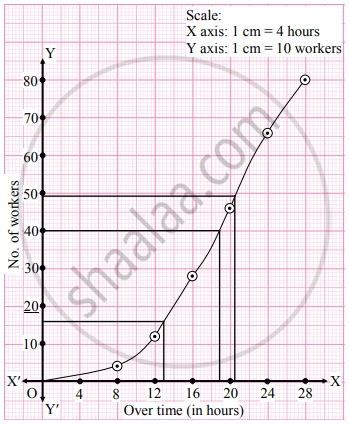Advertisement Remove all ads

# The following is the frequency distribution of overtime (per week) performed by various workers from a certain company. Determine the values of D2, Q2, and P61 graphically. - Mathematics and Statistics

Graph
Sum

The following is the frequency distribution of overtime (per week) performed by various workers from a certain company.

Determine the values of D2, Q2, and P61 graphically.

 Overtime(in hours) Below 8 8 – 12 12 – 16 16 – 20 20 – 24 24 and above No. of workers 4 8 16 18 20 14
Advertisement Remove all ads

#### Solution

To draw a ogive curve, we construct a less than cumulative frequency table as given below:

 Overtime (in hours) No. of workers (f) Less than cumulative frequency(c.f.) Below 8 4 4 8 – 12 8 12 12 – 16 16 28 16 – 20 18 46 20 –  24 20 66 24 and above 14 80 Total 80

Points to be plotted are (8, 4), (12, 12), (16, 28), (20, 46), (24, 66) and (28, 80)
Here, N = 80For D2, we have to consider (2"N")/(10)=(2xx80)/10 = 16

For Q2, we have to consider "N"/(2)=80/2 = 40

and for P61, we have to consider (61"N")/(100) = (61xx80)/(100) = 48..8
∴ We consider the values 16, 40, and 48.8 on the Y-axis. From these points, we draw the lines which are parallel to the X-axis. From the points where they intersect the less than ogive, we draw perpendiculars to X-axis. The values at the foot of perpendiculars represent the values of D2, Q2, and P61 respectively.

∴ D2 ≈ 13, Q2 ≈ 19, P61 ≈ 20.5

Concept: Graphical Location of Partition Values
Is there an error in this question or solution?
Advertisement Remove all ads

#### APPEARS IN

Advertisement Remove all ads
Advertisement Remove all ads
Share
Notifications

View all notifications

Forgot password?